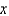Psychology

## Quiz 10 : Insulin AdministrationLooking for Drugs and Behavior Homework Help?# Quiz 10 : Insulin Administration

Give 17 units of insulin based on the sliding scale.

120 Have Want to Have 100 units : 1000 mL :: 12 units :mL 100= 1000 12 = 12,000= 120 mL per hr Proof: 12 1000 = 12,000 100 120 = 12,000

a. 10 Have Want to Have 5 units : 1 hour :: 50 units :hours 5= 50= 10 hours Proof: 1 50 = 50 5 10 = 50 b. 250 Have Want to Have 250 mL: 10 hours ::mL : 1 hour 10= 250= 25 mL per hr Proof: 250 1 = 250 10 25 = 250